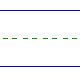Name: ______________________________________________ Date: _____________

Instructions: Find the sum.

 2 + 7 =3 + 4 =4 + 6 =1 + 6 =0 + 6 =2 + 5 =5 + 1 =2 + 6 =3 + 0 =7 + 2 =0 + 8 =6 + 1 =7 + 3 =0 + 3 =1 + 9 =1 + 2 =8 + 1 =0 + 2 =